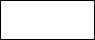﻿ Security light softwareHome//Security Lamp control by light level for Trinket

//18.12.14

//Program start

//Lamp and status LED off until light level falls.

//Lamp on for fixed period

//Lamp continues on for random period together with LED

//Lamp off for fixed period, LED still on

//LED flashes while light level rises above threshold

//Back to start

//analog pin designations

int readSensorpin = 1;  //Anolog pin is “1”

//digital pin designations

int ledPin = 1;

int sensorPin = 2;   //But to set it as input uses “2”

int lampPin = 0;

//variables

int lightValue;

long lvTotal;

long timeThen;

long timeNow;

long timeSince;

//constants

int downTime = 300;   //time to wait before unit starts again in minutes

long testing = 5000;  //10 secs in milli secs

int hour = 1800000;  //1 hour in milli secs

int dayLight = 35;  //light needs to rise above this to reset device

int lightThreshold = 8;

//was 13 in kitchen. 10 too high in hall?

int timeOn = 300;         // in minutes

void setup()

{

pinMode(sensorPin , INPUT);

pinMode(lampPin , OUTPUT);

pinMode(ledPin , OUTPUT);

analogWrite(lampPin , 0);   //lamp is off

}

void loop()

{

digitalWrite(ledPin , LOW);   //led is off

//wait for light level to fall

do

{

lvTotal = 0;

lightValue = 0;

for(int x = 0; x < 100; x++){

lvTotal  = lvTotal + lightValue;

delay(500);     //5 for testing, normally 600

}

lvTotal = lvTotal / 100;   //average light value

}while (lvTotal > 12 );

//turn on lamp

for(int x = 0; x < 256; x++){

analogWrite(lampPin, x);

delay(100);

}

//leave light on for a long time

timeThen = millis();

do

{

timeNow = millis();

timeSince = timeNow - timeThen;

}while (timeSince < 200 * 60000); // 60000 seems to be 2 minutes?

// delay random time up to 1 hour for testing = 10 sec

digitalWrite(ledPin , HIGH);   //led on

long rndTime = random(0, 1800000);

delay(rndTime);

//digitalWrite(ledPin , LOW);  //led off

// turn off light

for(int x = 255; x > -1 ; x--){

analogWrite(lampPin, x);

delay(5);

}

//wait for several hours

//led still on

timeThen = millis();

do

{

timeNow = millis();

timeSince = timeNow - timeThen;

}while (timeSince < 200 * 60000);  //downtime in minutes

//wait for light level to rise

do

{

lvTotal = 0;

lightValue = 0;

for(int x = 0; x < 100; x++){

lvTotal  = lvTotal + lightValue;

digitalWrite(ledPin , HIGH);

delay(300);

digitalWrite(ledPin , LOW);

delay(300);

}

lvTotal = lvTotal / 100;  // average light value

}while (lvTotal < 35 );

// originally < 30

}

Software for the Adafruit Trinket powered security light